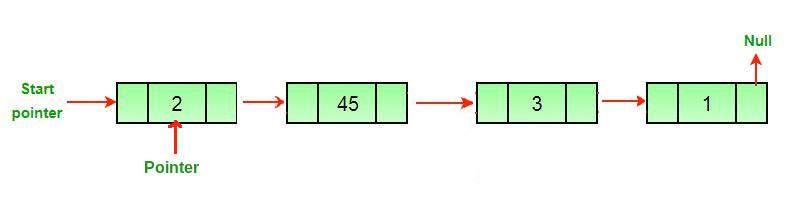Related Articles
Remove last node of the linked list
• Difficulty Level : Medium
• Last Updated : 19 Mar, 2020

Examples:

```Input: 1 -> 2 -> 3 -> 4 -> 5 -> NULL
Output: 1 -> 2 -> 3 -> 4 -> NULL

Explanation: The last node of the linked list
is 5, so 5 is deleted.

Input: 2 -> 4 -> 6 -> 8 -> 33 -> 67 -> NULL
Output: 2 -> 4 -> 6 -> 8 -> 33 -> NULL

Explanation: The last node of the linked list
is 67, so 67 is deleted.
```

## Recommended: Please try your approach on {IDE} first, before moving on to the solution.

Approach: To delete the last node of a linked list, find the second last node and make the next pointer of that node null.Algorithm:

1. If the first node is null or there is only one node, then return null
```if headNode == null then return null
if headNode.nextNode == null then free
```
2. Create an extra space secondLast, and traverse the linked list till the second last node.
```while secondLast.nextNode.nextNode != null
secondLast = secondLast.nextNode
```
3. delete the last node, i.e. the next node of second last node delete(secondLast.nextNode),and set the value of next of second last node to null.

Implementation:

## C++

 `// CPP program to remove last node of``// linked list.``#include ``using` `namespace` `std;`` ` `/* Link list node */``struct` `Node {``    ``int` `data;``    ``struct` `Node* next;``};`` ` `/* Function to remove the last node  ``   ``of the linked list */``Node* removeLastNode(``struct` `Node* head)``{``    ``if` `(head == NULL)``        ``return` `NULL;`` ` `    ``if` `(head->next == NULL) {``        ``delete` `head;``        ``return` `NULL;``    ``}`` ` `    ``// Find the second last node``    ``Node* second_last = head;``    ``while` `(second_last->next->next != NULL)``        ``second_last = second_last->next;`` ` `    ``// Delete last node``    ``delete` `(second_last->next);`` ` `    ``// Change next of second last``    ``second_last->next = NULL;`` ` `    ``return` `head;``}`` ` `// Function to push node at head``void` `push(``struct` `Node** head_ref, ``int` `new_data)``{``    ``struct` `Node* new_node = ``new` `Node;``    ``new_node->data = new_data;``    ``new_node->next = (*head_ref);``    ``(*head_ref) = new_node;``}`` ` `// Driver code``int` `main()``{``    ``/* Start with the empty list */``    ``Node* head = NULL;`` ` `    ``/* Use push() function to construct   ``       ``the below list 8 -> 23 -> 11 -> 29 -> 12 */``    ``push(&head, 12);``    ``push(&head, 29);``    ``push(&head, 11);``    ``push(&head, 23);``    ``push(&head, 8);`` ` `    ``head = removeLastNode(head);``    ``for` `(Node* temp = head; temp != NULL; temp = temp->next)``        ``cout << temp->data << ``" "``;`` ` `    ``return` `0;``}`

## Java

 `// Java program to remove last node of``// linked list.``class` `GFG {`` ` `    ``// Link list node /``    ``static` `class` `Node {``        ``int` `data;``        ``Node next;``    ``};`` ` `    ``// Function to remove the last node``    ``// of the linked list /``    ``static` `Node removeLastNode(Node head)``    ``{``        ``if` `(head == ``null``)``            ``return` `null``;`` ` `        ``if` `(head.next == ``null``) {``            ``return` `null``;``        ``}`` ` `        ``// Find the second last node``        ``Node second_last = head;``        ``while` `(second_last.next.next != ``null``)``            ``second_last = second_last.next;`` ` `        ``// Change next of second last``        ``second_last.next = ``null``;`` ` `        ``return` `head;``    ``}`` ` `    ``// Function to push node at head``    ``static` `Node push(Node head_ref, ``int` `new_data)``    ``{``        ``Node new_node = ``new` `Node();``        ``new_node.data = new_data;``        ``new_node.next = (head_ref);``        ``(head_ref) = new_node;``        ``return` `head_ref;``    ``}`` ` `    ``// Driver code``    ``public` `static` `void` `main(String args[])``    ``{``        ``// Start with the empty list /``        ``Node head = ``null``;`` ` `        ``// Use push() function to con``        ``// the below list 8 . 23 . 11 . 29 . 12 /``        ``head = push(head, ``12``);``        ``head = push(head, ``29``);``        ``head = push(head, ``11``);``        ``head = push(head, ``23``);``        ``head = push(head, ``8``);`` ` `        ``head = removeLastNode(head);``        ``for` `(Node temp = head; temp != ``null``; temp = temp.next)``            ``System.out.print(temp.data + ``" "``);``    ``}``}`` ` `// This code is contributed by Arnab Kundu`

## Python3

 `# Python3 program to remove the last node of ``# linked list.``import` `sys``import` `math`` ` `# Link list node``class` `Node:``    ``def` `__init__(``self``, data):``        ``self``.data ``=` `data``        ``self``.``next` `=` `None`` ` `# Function to push node at head ``def` `push(head, data):``    ``if` `not` `head:``        ``return` `Node(data)``    ``temp ``=` `Node(data)``    ``temp.``next` `=` `head``    ``head ``=` `temp``    ``return` `head`` ` `# Function to remove the last node ``# of the linked list``def` `removeLastNode(head):``    ``if` `head ``=``=` `None``:``        ``return` `None``    ``if` `head.``next` `=``=` `None``:``        ``head ``=` `None``        ``return` `None``    ``second_last ``=` `head``    ``while``(second_last.``next``.``next``):``        ``second_last ``=` `second_last.``next``    ``second_last.``next` `=` `None``    ``return` `head`` ` `# Driver code``if` `__name__``=``=``'__main__'``:`` ` `    ``# Start with the empty list``    ``head ``=` `None``    ``# Use push() function to con ``    ``# the below list 8 . 23 . 11 . 29 . 12 ``    ``head ``=` `push(head, ``12``)``    ``head ``=` `push(head, ``29``)``    ``head ``=` `push(head, ``11``)``    ``head ``=` `push(head, ``23``)``    ``head ``=` `push(head, ``8``)`` ` `    ``head ``=` `removeLastNode(head)``    ``while``(head):``        ``print``(``"{} "``.``format``(head.data), end ``=``"")``        ``head ``=` `head.``next`` ` `# This code is contributed by Vikash kumar 37`

## C#

 `// C# program to remove last node of``// linked list.``using` `System;`` ` `class` `GFG {`` ` `    ``// Link list node /``    ``public` `class` `Node {``        ``public` `int` `data;``        ``public` `Node next;``    ``};`` ` `    ``// Function to remove the last node``    ``// of the linked list /``    ``static` `Node removeLastNode(Node head)``    ``{``        ``if` `(head == ``null``)``            ``return` `null``;`` ` `        ``if` `(head.next == ``null``) {``            ``return` `null``;``        ``}`` ` `        ``// Find the second last node``        ``Node second_last = head;``        ``while` `(second_last.next.next != ``null``)``            ``second_last = second_last.next;`` ` `        ``// Change next of second last``        ``second_last.next = ``null``;`` ` `        ``return` `head;``    ``}`` ` `    ``// Function to push node at head``    ``static` `Node push(Node head_ref, ``int` `new_data)``    ``{``        ``Node new_node = ``new` `Node();``        ``new_node.data = new_data;``        ``new_node.next = (head_ref);``        ``(head_ref) = new_node;``        ``return` `head_ref;``    ``}`` ` `    ``// Driver code``    ``public` `static` `void` `Main(String[] args)``    ``{``        ``// Start with the empty list /``        ``Node head = ``null``;`` ` `        ``// Use push() function to con``        ``// the below list 8 . 23 . 11 . 29 . 12 /``        ``head = push(head, 12);``        ``head = push(head, 29);``        ``head = push(head, 11);``        ``head = push(head, 23);``        ``head = push(head, 8);`` ` `        ``head = removeLastNode(head);``        ``for` `(Node temp = head; temp != ``null``; temp = temp.next)``            ``Console.Write(temp.data + ``" "``);``    ``}``}`` ` `/* This code contributed by PrinciRaj1992 */`
Output:
```8 23 11 29
```

Complexity Analysis:

• Time Complexity: O(n).
The algorithm involves traversal of the linked list till its end, So the time complexity required is O(n).
• Space Complexity: O(1).
No extra space is required, so the space complexity is constant

Attention reader! Don’t stop learning now. Get hold of all the important DSA concepts with the DSA Self Paced Course at a student-friendly price and become industry ready.  To complete your preparation from learning a language to DS Algo and many more,  please refer Complete Interview Preparation Course.

In case you wish to attend live classes with industry experts, please refer Geeks Classes Live and Geeks Classes Live USA

My Personal Notes arrow_drop_up